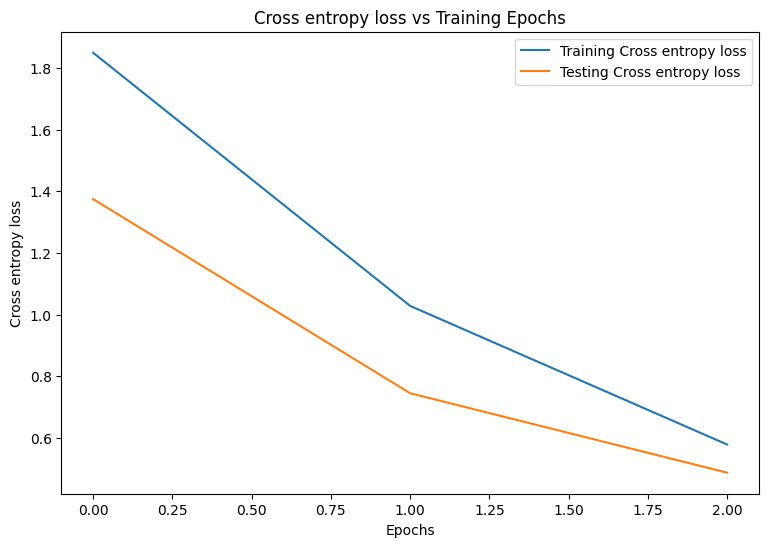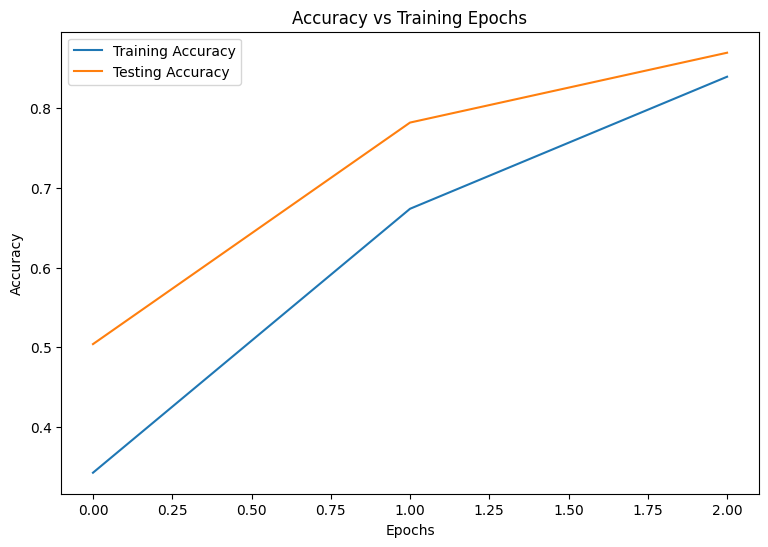Thanks for tuning in to Google I/O. View all sessions on demand

# Distributed training with Core APIs and DTensor

## Introduction

This notebook uses the TensorFlow Core low-level APIs and DTensor to demonstrate a data parallel distributed training example. Visit the Core APIs overview to learn more about TensorFlow Core and its intended use cases. Refer to the DTensor Overview guide and Distributed Training with DTensors tutorial to learn more about DTensor.

This example uses the same model and optimizer shown in the multilayer perceptrons tutorial. See this tutorial first to get comfortable with writing an end-to-end machine learning workflow with the Core APIs.

## Overview of data parallel training with DTensor

Before building an MLP that supports distribution, take a moment to explore the fundamentals of DTensor for data parallel training.

DTensor allows you to run distributed training across devices to improve efficiency, reliability and scalability. DTensor distributes the program and tensors according to the sharding directives through a procedure called Single program, multiple data (SPMD) expansion. A variable of a `DTensor` aware layer is created as `dtensor.DVariable`, and the constructors of `DTensor` aware layer objects take additional `Layout` inputs in addition to the usual layer parameters.

The main ideas for data parallel training are as follows:

• Model variables are replicated on N devices each.
• A global batch is split into N per-replica batches.
• Each per-replica batch is trained on the replica device.
• The gradient is reduced before weight up data is collectively performed on all replicas.
• Data parallel training provides nearly linear speed with respect to the number of devices

## Setup

DTensor is part of TensorFlow 2.9.0 release.

``````#!pip install --quiet --upgrade --pre tensorflow
``````
``````import matplotlib
from matplotlib import pyplot as plt
# Preset Matplotlib figure sizes.
matplotlib.rcParams['figure.figsize'] = [9, 6]
``````
``````import tensorflow as tf
import tensorflow_datasets as tfds
from tensorflow.experimental import dtensor
print(tf.__version__)
# Set random seed for reproducible results
tf.random.set_seed(22)
``````
```2022-12-14 02:39:44.054897: W tensorflow/compiler/xla/stream_executor/platform/default/dso_loader.cc:64] Could not load dynamic library 'libnvinfer.so.7'; dlerror: libnvinfer.so.7: cannot open shared object file: No such file or directory
2022-12-14 02:39:44.054995: W tensorflow/compiler/xla/stream_executor/platform/default/dso_loader.cc:64] Could not load dynamic library 'libnvinfer_plugin.so.7'; dlerror: libnvinfer_plugin.so.7: cannot open shared object file: No such file or directory
2022-12-14 02:39:44.055005: W tensorflow/compiler/tf2tensorrt/utils/py_utils.cc:38] TF-TRT Warning: Cannot dlopen some TensorRT libraries. If you would like to use Nvidia GPU with TensorRT, please make sure the missing libraries mentioned above are installed properly.
2.11.0
```

Configure 8 virtual CPUs for this experiment. DTensor can also be used with GPU or TPU devices. Given that this notebook uses virtual devices, the speedup gained from distributed training is not noticeable.

``````def configure_virtual_cpus(ncpu):
phy_devices = tf.config.list_physical_devices('CPU')
tf.config.set_logical_device_configuration(phy_devices, [
tf.config.LogicalDeviceConfiguration(),
] * ncpu)

configure_virtual_cpus(8)

DEVICES = [f'CPU:{i}' for i in range(8)]
devices = tf.config.list_logical_devices('CPU')
device_names = [d.name for d in devices]
device_names
``````
```['/device:CPU:0',
'/device:CPU:1',
'/device:CPU:2',
'/device:CPU:3',
'/device:CPU:4',
'/device:CPU:5',
'/device:CPU:6',
'/device:CPU:7']
```

## The MNIST Dataset

The dataset is available from TensorFlow Datasets. Split the data into training and testing sets. Only use 5000 examples for training and testing to save time.

``````train_data, test_data = tfds.load("mnist", split=['train[:5000]', 'test[:5000]'], batch_size=128, as_supervised=True)
``````

### Preprocessing the data

Preprocess the data by reshaping it to be 2-dimensional and by rescaling it to fit into the unit interval, [0,1].

``````def preprocess(x, y):
# Reshaping the data
x = tf.reshape(x, shape=[-1, 784])
# Rescaling the data
x = x/255
return x, y

train_data, test_data = train_data.map(preprocess), test_data.map(preprocess)
``````

## Build the MLP

Build an MLP model with DTensor aware layers.

### The dense layer

Start by creating a dense layer module that supports DTensor. The `dtensor.call_with_layout` function can be used to call a function that takes in a DTensor input and produces a DTensor output. This is useful for initializing a DTensor variable, `dtensor.DVariable`, with a TensorFlow supported function.

``````class DenseLayer(tf.Module):

def __init__(self, in_dim, out_dim, weight_layout, activation=tf.identity):
super().__init__()
# Initialize dimensions and the activation function
self.in_dim, self.out_dim = in_dim, out_dim
self.activation = activation

# Initialize the DTensor weights using the Xavier scheme
uniform_initializer = tf.function(tf.random.stateless_uniform)
xavier_lim = tf.sqrt(6.)/tf.sqrt(tf.cast(self.in_dim + self.out_dim, tf.float32))
self.w = dtensor.DVariable(
dtensor.call_with_layout(
uniform_initializer, weight_layout,
shape=(self.in_dim, self.out_dim), seed=(22, 23),
minval=-xavier_lim, maxval=xavier_lim))

# Initialize the bias with the zeros
bias_layout = weight_layout.delete()
self.b = dtensor.DVariable(
dtensor.call_with_layout(tf.zeros, bias_layout, shape=[out_dim]))

def __call__(self, x):
# Compute the forward pass
return self.activation(z)
``````

### The MLP sequential model

Now create an MLP module that executes the dense layers sequentially.

``````class MLP(tf.Module):

def __init__(self, layers):
self.layers = layers

def __call__(self, x, preds=False):
# Execute the model's layers sequentially
for layer in self.layers:
x = layer(x)
return x
``````

Performing "data-parallel" training with DTensor is equivalent to `tf.distribute.MirroredStrategy`. To do this each device will run the same model on a shard of the data batch. So you'll need the following:

Create a DTensor mesh that consists of a single batch dimension, where each device becomes a replica that receives a shard from the global batch. Use this mesh to instantiate an MLP mode with the following architecture:

Forward Pass: ReLU(784 x 700) x ReLU(700 x 500) x Softmax(500 x 10)

``````mesh = dtensor.create_mesh([("batch", 8)], devices=DEVICES)
weight_layout = dtensor.Layout([dtensor.UNSHARDED, dtensor.UNSHARDED], mesh)

input_size = 784
hidden_layer_1_size = 700
hidden_layer_2_size = 500
hidden_layer_2_size = 10

mlp_model = MLP([
DenseLayer(in_dim=input_size, out_dim=hidden_layer_1_size,
weight_layout=weight_layout,
activation=tf.nn.relu),
DenseLayer(in_dim=hidden_layer_1_size , out_dim=hidden_layer_2_size,
weight_layout=weight_layout,
activation=tf.nn.relu),
DenseLayer(in_dim=hidden_layer_2_size, out_dim=hidden_layer_2_size,
weight_layout=weight_layout)])
``````

### Training metrics

Use the cross-entropy loss function and accuracy metric for training.

``````def cross_entropy_loss(y_pred, y):
# Compute cross entropy loss with a sparse operation
sparse_ce = tf.nn.sparse_softmax_cross_entropy_with_logits(labels=y, logits=y_pred)
return tf.reduce_mean(sparse_ce)

def accuracy(y_pred, y):
# Compute accuracy after extracting class predictions
class_preds = tf.argmax(y_pred, axis=1)
is_equal = tf.equal(y, class_preds)
return tf.reduce_mean(tf.cast(is_equal, tf.float32))
``````

### Optimizer

Using an optimizer can result in significantly faster convergence compared to standard gradient descent. The Adam optimizer is implemented below and has been configured to be compatible with DTensor. In order to use Keras optimizers with DTensor, refer to the experimental`tf.keras.dtensor.experimental.optimizers` module.

``````class Adam(tf.Module):

def __init__(self, model_vars, learning_rate=1e-3, beta_1=0.9, beta_2=0.999, ep=1e-7):
# Initialize optimizer parameters and variable slots
self.model_vars = model_vars
self.beta_1 = beta_1
self.beta_2 = beta_2
self.learning_rate = learning_rate
self.ep = ep
self.t = 1.
self.v_dvar, self.s_dvar = [], []
# Initialize optimizer variable slots
for var in model_vars:
v = dtensor.DVariable(dtensor.call_with_layout(tf.zeros, var.layout, shape=var.shape))
s = dtensor.DVariable(dtensor.call_with_layout(tf.zeros, var.layout, shape=var.shape))
self.v_dvar.append(v)
self.s_dvar.append(s)

# Update the model variables given their gradients
for i, (d_var, var) in enumerate(zip(grads, self.model_vars)):
self.v_dvar[i].assign(self.beta_1*self.v_dvar[i] + (1-self.beta_1)*d_var)
self.s_dvar[i].assign(self.beta_2*self.s_dvar[i] + (1-self.beta_2)*tf.square(d_var))
v_dvar_bc = self.v_dvar[i]/(1-(self.beta_1**self.t))
s_dvar_bc = self.s_dvar[i]/(1-(self.beta_2**self.t))
var.assign_sub(self.learning_rate*(v_dvar_bc/(tf.sqrt(s_dvar_bc) + self.ep)))
self.t += 1.
return
``````

### Data packing

Start by writing a helper function for transferring data to the device. This function should use `dtensor.pack` to send (and only send) the shard of the global batch that is intended for a replica to the device backing the replica. For simplicity, assume a single-client application.

Next, write a function that uses this helper function to pack the training data batches into DTensors sharded along the batch (first) axis. This ensures that DTensor evenly distributes the training data to the 'batch' mesh dimension. Note that in DTensor, the batch size always refers to the global batch size; therefore, the batch size should be chosen such that it can be divided evenly by the size of the batch mesh dimension. Additional DTensor APIs to simplify `tf.data` integration are planned, so please stay tuned.

``````def repack_local_tensor(x, layout):
# Repacks a local Tensor-like to a DTensor with layout
# This function assumes a single-client application
x = tf.convert_to_tensor(x)
sharded_dims = []

# For every sharded dimension, use tf.split to split the along the dimension.
# The result is a nested list of split-tensors in queue.
queue = [x]
for axis, dim in enumerate(layout.sharding_specs):
if dim == dtensor.UNSHARDED:
continue
num_splits = layout.shape[axis]
queue = tf.nest.map_structure(lambda x: tf.split(x, num_splits, axis=axis), queue)
sharded_dims.append(dim)

# Now we can build the list of component tensors by looking up the location in
# the nested list of split-tensors created in queue.
components = []
for locations in layout.mesh.local_device_locations():
t = queue
for dim in sharded_dims:
split_index = locations[dim]  # Only valid on single-client mesh.
t = t[split_index]
components.append(t)

return dtensor.pack(components, layout)

def repack_batch(x, y, mesh):
# Pack training data batches into DTensors along the batch axis
x = repack_local_tensor(x, layout=dtensor.Layout(['batch', dtensor.UNSHARDED], mesh))
y = repack_local_tensor(y, layout=dtensor.Layout(['batch'], mesh))
return x, y
``````

### Training

Write a traceable function that executes a single training step given a batch of data. This function does not require any special DTensor annotations. Also write a function that executes a test step and returns the appropriate performance metrics.

``````@tf.function
def train_step(model, x_batch, y_batch, loss, metric, optimizer):
# Execute a single training step
y_pred = model(x_batch)
batch_loss = loss(y_pred, y_batch)
# Compute gradients and update the model's parameters
# Return batch loss and accuracy
batch_acc = metric(y_pred, y_batch)
return batch_loss, batch_acc

@tf.function
def test_step(model, x_batch, y_batch, loss, metric):
# Execute a single testing step
y_pred = model(x_batch)
batch_loss = loss(y_pred, y_batch)
batch_acc = metric(y_pred, y_batch)
return batch_loss, batch_acc
``````

Now, train the MLP model for 3 epochs with a batch size of 128.

``````# Initialize the training loop parameters and structures
epochs = 3
batch_size = 128
train_losses, test_losses = [], []
train_accs, test_accs = [], []

# Format training loop
for epoch in range(epochs):
batch_losses_train, batch_accs_train = [], []
batch_losses_test, batch_accs_test = [], []

# Iterate through training data
for x_batch, y_batch in train_data:
x_batch, y_batch = repack_batch(x_batch, y_batch, mesh)
batch_loss, batch_acc = train_step(mlp_model, x_batch, y_batch, cross_entropy_loss, accuracy, optimizer)
# Keep track of batch-level training performance
batch_losses_train.append(batch_loss)
batch_accs_train.append(batch_acc)

# Iterate through testing data
for x_batch, y_batch in test_data:
x_batch, y_batch = repack_batch(x_batch, y_batch, mesh)
batch_loss, batch_acc = test_step(mlp_model, x_batch, y_batch, cross_entropy_loss, accuracy)
# Keep track of batch-level testing
batch_losses_test.append(batch_loss)
batch_accs_test.append(batch_acc)

# Keep track of epoch-level model performance
train_loss, train_acc = tf.reduce_mean(batch_losses_train), tf.reduce_mean(batch_accs_train)
test_loss, test_acc = tf.reduce_mean(batch_losses_test), tf.reduce_mean(batch_accs_test)
train_losses.append(train_loss)
train_accs.append(train_acc)
test_losses.append(test_loss)
test_accs.append(test_acc)
print(f"Epoch: {epoch}")
print(f"Training loss: {train_loss.numpy():.3f}, Training accuracy: {train_acc.numpy():.3f}")
print(f"Testing loss: {test_loss.numpy():.3f}, Testing accuracy: {test_acc.numpy():.3f}")
``````
```Epoch: 0
Training loss: 1.850, Training accuracy: 0.343
Testing loss: 1.375, Testing accuracy: 0.504
Epoch: 1
Training loss: 1.028, Training accuracy: 0.674
Testing loss: 0.744, Testing accuracy: 0.782
Epoch: 2
Training loss: 0.578, Training accuracy: 0.839
Testing loss: 0.486, Testing accuracy: 0.869
```

### Performance evaluation

Start by writing a plotting function to visualize the model's loss and accuracy during training.

``````def plot_metrics(train_metric, test_metric, metric_type):
# Visualize metrics vs training Epochs
plt.figure()
plt.plot(range(len(train_metric)), train_metric, label = f"Training {metric_type}")
plt.plot(range(len(test_metric)), test_metric, label = f"Testing {metric_type}")
plt.xlabel("Epochs")
plt.ylabel(metric_type)
plt.legend()
plt.title(f"{metric_type} vs Training Epochs");
``````
``````plot_metrics(train_losses, test_losses, "Cross entropy loss")
````````````plot_metrics(train_accs, test_accs, "Accuracy")
``````The integration of `tf.saved_model` and DTensor is still under development. As of TensorFlow 2.9.0, tf.saved_model only accepts DTensor models with fully replicated variables. As a workaround, you can convert a DTensor model to a fully replicated one by reloading a checkpoint. However, after a model is saved, all DTensor annotations are lost and the saved signatures can only be used with regular Tensors. This tutorial will be updated to showcase the integration once it is solidified.